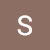# Statistics for Data Science — Part IV: Hypothesis Testing

This article is the fourth and final part of a series and I will cover hypotheses testing. In the previous article, statistical inference was defined as the second major branch of statistics and also very important for the data scientist. The target was defined as making more meaningful estimates by specifying an interval of values on a number line, together with a statement of how confident you are that your interval contains the population parameter.

You…

# ML Regression Analysis, Implemented on Streamlit, Deployed on AWS EC2

In a previous article, I got quite satisfactory results using various machine learning regression algorithms in estimating the compressive strength values of concrete using 8 different parameters. I wrote a follow-up to this article and applied deep learning to the same data set and compared the performances.

In this article I am going to give the details about the steps involved in implementing a Machine Learning Regression Analysis on Streamlit, followed by deploying on AWS EC2.

Starting with definitions, Streamlit is an open-source Python library that makes it easy to generate and share beautiful, custom web apps for machine learning…

# Statistics for Data Science — Part III: Statistical Inference

This article is a third of a series and I will cover the parts of probability that are related to data science. Statistical inference is defined as the second major branch of statistics and very important for the data scientist. The target will be making more meaningful estimates by specifying an interval of values on a number line, together with a statement of how confident you are that your interval contains the population parameter.

I will try to give information about the following subjects:

· Central Limit Theorem

· Confidence Intervals,

You may find the first article of this series…

# Statistics for Data Science — Part II: Probability

This article is a second of a series and I will cover the parts of probability that are related to data science. Probability is very important for the data scientist and I will try to answer the following questions:

· Basic Concepts of Probability,

· Probability Distributions.

You may find the first article of this series here.

Types of Probability

Once again, let us start with the Wikipedia definition for probability: “Probability is the branch of mathematics concerning numerical descriptions of how likely an event is to occur, or how likely it is that a proposition is true. The probability…

# Statistics for Data Science — Part I: Introduction

In 1970s, John Tukey produced a new definition for statistics; instead of calling it a pure mathematical science, he suggested that deriving hypotheses from data was the future. It was a reform of statistics and announcement of an as-yet unrecognized science. It has been called Data Science for a long time and it is influenced by computer science, mathematics, statistics as well as the applied sciences.

In this series of articles, I will cover the basic parts of statistics which are crucial for a data scientist and I will try to answer the following questions:

· What is statistics?

·…

# Deep Learning or Machine Learning: That is the Question

This is a follow-up to my previous article: Comparison of Regression Analysis Algorithms. I applied Deep Learning and compared the results with the performances of the Machine Learning algorithms in the above-mentioned article.

In the previous article, I got quite satisfactory results using various machine learning regression algorithms in estimating the compressive strength values of concrete using 8 different parameters. Regression analysis may be defined as a type of predictive modelling technique which investigates the relationship between a dependent (target) and independent variable(s) (predictor). …

# Analysis of F-16 Accidents by NLP

Gursev Pirge and Alp Pirge

This article involves the use of Natural Language Processing (NLP), with the target of analyzing the causes of the F-16 fighter aircraft accidents and incidents between 1979 and 2019 in the US Air Force (USAF). We used the data set provided by F-16.net, which gave us the dates, type and the accident report. In the previous paper and in the very first paper, the analysis focused on both civilian and military aircraft. In this study, we decided to focus on the F-16 Fighting Falcon, which is operated by 26 air forces around the world since…

# Aircraft Crash Analysis by Word Cloud

This article involves the use of Natural Language Processing (NLP), with the target of analyzing the causes of airplane accidents between 1969 and 2009. We used the data set provided by data.world, which is a detailed database about the airplane crashes and gives the opportunity to make an in-depth analysis for anyone interested in the subject. As it was mentioned in the previous paper, the data started from 1908, but we decided to analyze the modern era of flight in order to reflect the effectiveness of the modern-day aerospace safety standards.

Wikipedia’s definition is: “Natural language processing (NLP) is a…

# Performance Comparison of Multi-Class Classification Algorithms

This article comprises the application and comparison of supervised multi-class classification algorithms to a dataset, which involves the chemical compositions (features) and types (four major types — target) of stainless steels. The dataset is quite small in numbers, but very accurate.

Stainless steel alloy datasets are commonly limited in size, thus restraining applications of Machine Learning (ML) techniques for classification. I explored the potential of 6 different classification algorithms, in the context of a small dataset of 62 samples, for outcome prediction in type classification.

In this article, multi-class classification was analyzed using various algorithms, with the target of classifying…

# Comparison of Regression Analysis Algorithms